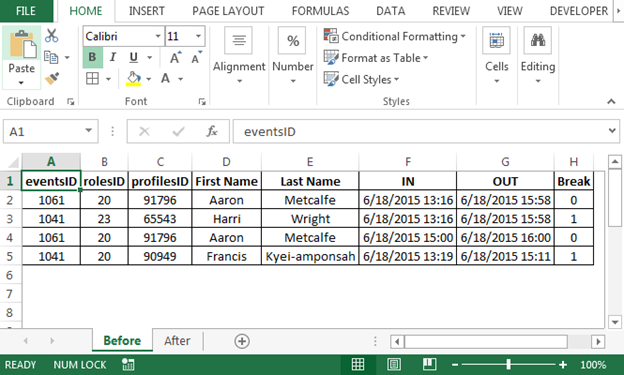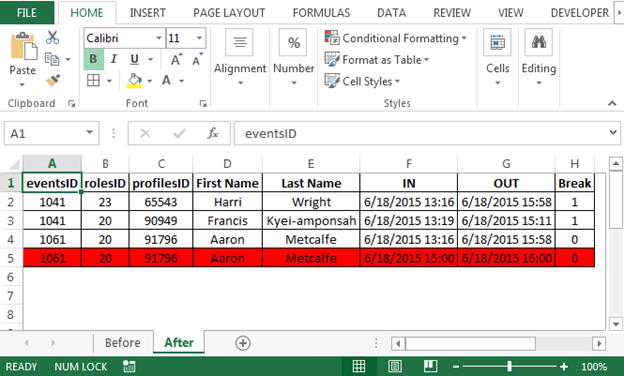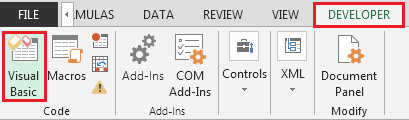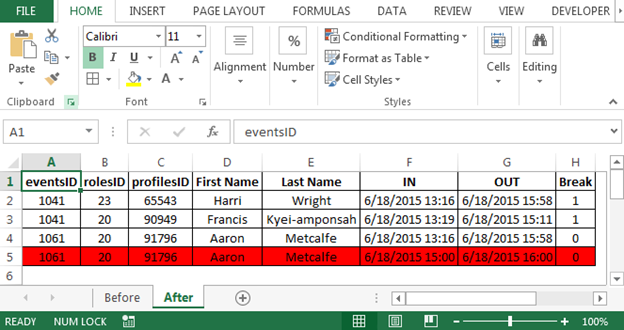# Identifying duplicate time frames

If you want to find out the duplicate row having multiple entries for same person & you want to find out duplicate entries, then you should not miss this article. We will provide a macro code to help you identifying the duplicate rows. In this article, we will learn how to find duplicated rows based on particular column.

Question: I have a spreadsheet with multiple time stamped entries for people. These people can clock in or out in several places at the same time. Trying to write a formula or macro (not sure which achieves the aim best in this case) that will search the data and highlight in red lines with times that overlap for a specific person. Is this possible and is it something somebody could help with please? Many thanks.

The original question can be found here

Following is the snapshot of before sheet:Following is the snapshot of after sheet:To get the code; we need to follow the below steps to launch Visual Basic editor screen

• Click on Developer tab
• From Code group, select Visual Basic• Copy the below code in the standard module

```Sub FindOverlapTime()
Dim rng As Range, cell As Range, trng As Range, tcell As Range
Dim lr As Long

lr = Cells(Rows.Count, "A").End(xlUp).Row
Range("A2:H" & lr).Interior.ColorIndex = xlNone
Set rng = Range("C2:C" & lr)
For Each cell In rng
If Application.CountIf(Range("C2", cell), cell.Value) > 1 Then
Set trng = Range("F2:F" & cell.Row - 1)
For Each tcell In trng
If tcell.Offset(0, -3) = cell Then
If (cell.Offset(0, 3) >= tcell And cell.Offset(0, 3) <= tcell.Offset(0, 1)) _                 Or (cell.Offset(0, 4) >= tcell And cell.Offset(0, 4) <= tcell.Offset(0, 1)) Then
Range("A" & cell.Row & ":H" & cell.Row).Interior.ColorIndex = 3
End If
End If
Next tcell
End If
Next cell
End Sub
```
• As we run the macro, we will get the result; refer below snapshot:Code Explanation:

• Declare rng, cell, trng, tcell as range
• Lr as long
• lr = Cells(Rows.Count, "A").End(xlUp).Row will check the last row of the current sheet.
• Range("A2:H" & lr).Interior.ColorIndex = xlNone; this will select range starting from cell A2 to H column till the last row (A2:H5 will be selected in our example) and will ensure no color is filled.
• Set rng = Range("C2:C" & lr); column C (Profiles ID) will be stored in rng
• For Each cell In rng; now we will run For each loop in rng i.e. column C
• If Application.CountIf(Range("C2", cell), cell.Value) > 1 Then; this will check the number of times the cell value is greater than 1; if found greater than 1, then
• Set trng = Range("F2:F" & cell.Row - 1); now we will set column F i.e. IN time in trng
• The next we will run For Each Loop in trng and check if there is any duplicate row and highlight it with red color if found one.

Conclusion: In this way, we can find duplicate values using macro code and can remove them later.